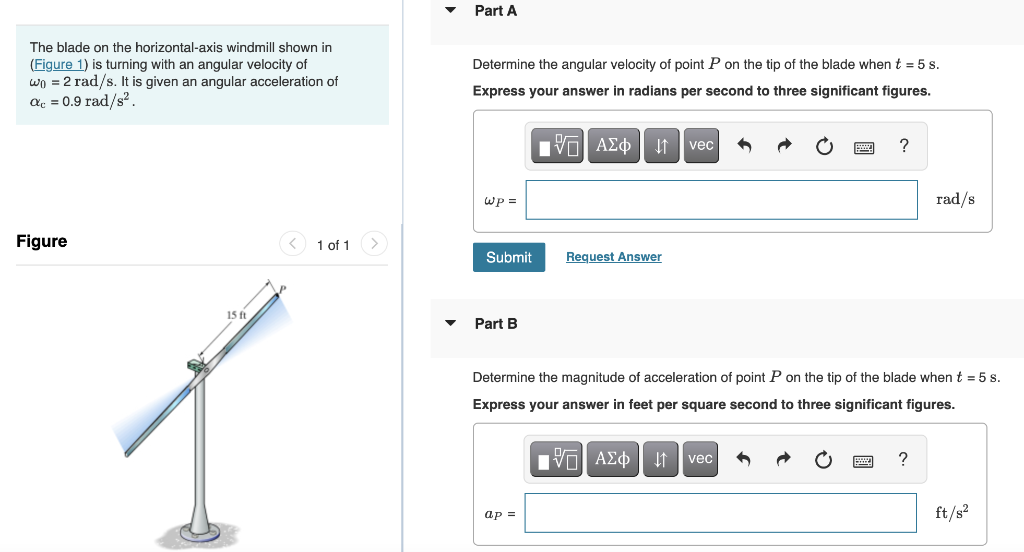# Question The blade on the horizontal-axis windmill shown in (Figure 1) is turning with an angular velocity of Determine the angular velocity of point $$P$$ on the tip of the blade when $$t=5 \mathrm{~s}$$. $$\omega_{0}=2 \mathrm{rad} / \mathrm{s}$$. It is given an angular acceleration of Express your answer in radians per second to three significant figures. \( The blade on the horizontal-axis windmill shown in (Figure 1) is turning with an angular velocity of Determine the angular velocity of point P on the tip of the blade when t=5 s. ω0​=2rad/s. It is given an angular acceleration of Express your answer in radians per second to three significant figures. αc​=0.9rad/s2 8 Figure 1 of 1 Part B Determine the magnitude of acceleration of point P on the tip of the blade when t=5 s. Express your answer in feet per square second to three significant figures.BZTQCF The Asker · Civil EngineeringTranscribed Image Text: The blade on the horizontal-axis windmill shown in (Figure 1) is turning with an angular velocity of Determine the angular velocity of point P on the tip of the blade when t=5 s. ω0​=2rad/s. It is given an angular acceleration of Express your answer in radians per second to three significant figures. αc​=0.9rad/s2 8 Figure 1 of 1 Part B Determine the magnitude of acceleration of point P on the tip of the blade when t=5 s. Express your answer in feet per square second to three significant figures.
More
Transcribed Image Text: The blade on the horizontal-axis windmill shown in (Figure 1) is turning with an angular velocity of Determine the angular velocity of point P on the tip of the blade when t=5 s. ω0​=2rad/s. It is given an angular acceleration of Express your answer in radians per second to three significant figures. αc​=0.9rad/s2 8 Figure 1 of 1 Part B Determine the magnitude of acceleration of point P on the tip of the blade when t=5 s. Express your answer in feet per square second to three significant figures.